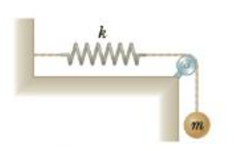Chapter 8, Problem 95AP

Chapter
Section
Textbook Problem

A 3.2-kg sphere is suspended by a cord that passes over a 1.8-kg pulley of radius 3.8 cm. The cord is attached to a spring whose force constant is k = 86 N/m as in figure P8.95. Assume the pulley is a solid disk, (a) If the sphere is released from rest with the spring unstretched, what distance does the sphere fall through before stopping? (b) Find the speed of the sphere after it has fallen 25 cm.Figure P8.95

(a)

To determine
The distance that the sphere fall through before stopping if the sphere is released from rest with the spring unstretched.

Explanation
From conservation of energy, the distance that the sphere fall before stopping it is calculated as follows and first assume that the ball starts at negative y axis of the coordinate such that KEr,f+KEt,f+PEg,f+PEs,f=KEr,i+KEt,i+PEg,i+PEs,i and initial and final translational speed of the sphere are zero and similarly, the initial and final angular speed of the pulley also be zero. 0+0+mgh+kh2/2=0+0+0+0 and by solving this, the distance is calculated.

Given info: The mass of the ball is 3.2kg , acceleration due to gravity is 9.80m/s2 , and the spring constant is 86N/m .

The formula for the distance that the sphere fall through before stopping if the sphere is released from rest with the spring unstretched is,

h=2mgk

• m is mass of the sphere

(b)

To determine
The speed of the sphere after it has fallen 25 cm.

Still sussing out bartleby?

Check out a sample textbook solution.

See a sample solution

The Solution to Your Study Problems

Bartleby provides explanations to thousands of textbook problems written by our experts, many with advanced degrees!

Get Started